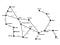# An overview in AI and path to Data Mining methods (part 3)

To get to know about data mining techniques we should have a base knowledge of Artificial Intelligence. Here I wrote about AI from the book ‘Artificial intelligence book by Peter Norvig and Stuart J. Russell’ to better understand how AI algorithms or specifically data mining algorithms work. I will write in four sections to understand easier.

in the previous sections, we studied what is AI and how environments and agents work. In this section, we would learn how to solve our problems with searching.

first of all, we need to know how to solve problems like a path direction problem.

# first step: Problem formulation

we need to formalize the problem, so our algorithm works for the problem. To formalize the problem we need to answer these five questions:

1. What is the first state? The initial point (state) of the agent.
2. What actions the agent can do? The set of possible actions our agent can do in response to the environment.
3. What is our successor function? The successor function shows the actions the agent can do in an example state of ‘X’.
4. What is our goal test function? We might choose a function to test each state whether is the goal we wanted or not. If the state is our goal state we would stop and finish the algorithm.
5. What is our Path cost function? Another function we might use in the algorithm is the Path cost function. Its duty is to calculate the path we’ve taken from the initial state to the goal or a specific state.

So the main thing here was to formulate our problem. The example below will help you more to understand what is it.

# Formalizing the famous Bucharest problem

The Bucharest problem is mentioned a lot in Russel's textbook, In this problem, we would like to find the minimum path from Arad to Bucharest. As you can see below the image is the map between different cities.image1. The map used in this problem taken from Russell's textbook. The numbers are costs to go from a city to another

So let’s formalize the problem.

1. First state: we’re in Arad.
2. Actions: Going from a city to another city.
3. Successor function: The function here shows available cities we can go from a city. output is a set of <actions, final_state> for example you can see below:

Successor(In_Arad) = { <Go(Zerilan), In_Zerilan>, <Go(Sibiu), In_Sibiu>, <Go(Timisora), In_Timisora> }

4. Goal test function: Test whether we arrived at Bucharest or not. you can see a pseudo code below ( x here is our state as input ).

`bool goal_test(x){   if(x == In_Bucharest) return true;   else return false;}`

5. Path cost function: show the cost from the initial state (Arad) to the present state. For example if we started from Arad and passed Timisoara and Lugoj and now we’re in the state of In_mehadia, the Path cost function will show the output 118+111+70.

So till now, we learned how to formalize our problems. It’s time to learn some searching algorithms.

# Searching Algorithms

first of all we need to mention that searching algorithms are divided into two groups:

1. Uninformed Search (Blind Search): In this type of algorithm, there is no information about the problem, and agents use brute-force search (agents would search everything possible).
2. Informed Search (Heuristic Search): This type of algorithm agent knows some information about the problem. For an example of the Bucharest problem, our agent knows which city is closer to its present state, or in general, they know which state is more promising.

## UnInformed Search Algorithms

http://chalmersgu-ai-course.github.io/AI-lecture-slides/lecture2.pdf

2. Depth-First Search (DFS)
3. Uniformed-Cost Search (UCS)
4. Depth-Limited Search (DLS)
5. Iterative-Deeping Search (IDS)
6. Iterative-Lengthening Search (ILS)
7. Bidirectional Search (BS)

## Informed Search Algorithms

For Informed Search Algorithms you can download this file to better understand them.

http://chalmersgu-ai-course.github.io/AI-lecture-slides/lecture3.pdf

1. A*
2. Iterative Deeping A*
3. Recursive Best First Search (RBFS)
4. Simplified Memory-Bounded A* (SMA*)
5. Local Search Algorithms

So until here, we learned how to formalize our problems and we know some algorithms for it. In the next and last section, we would learn how Local Search Algorithms work and specifically how we use them in data mining methods. I’ll publish the last part in a week.

Thanks for reading. Stay tuned :)

A senior student of computer engineering. Android application developer, And now learning AI and Data-Mining. My Github page link: https://github.com/amindadgar